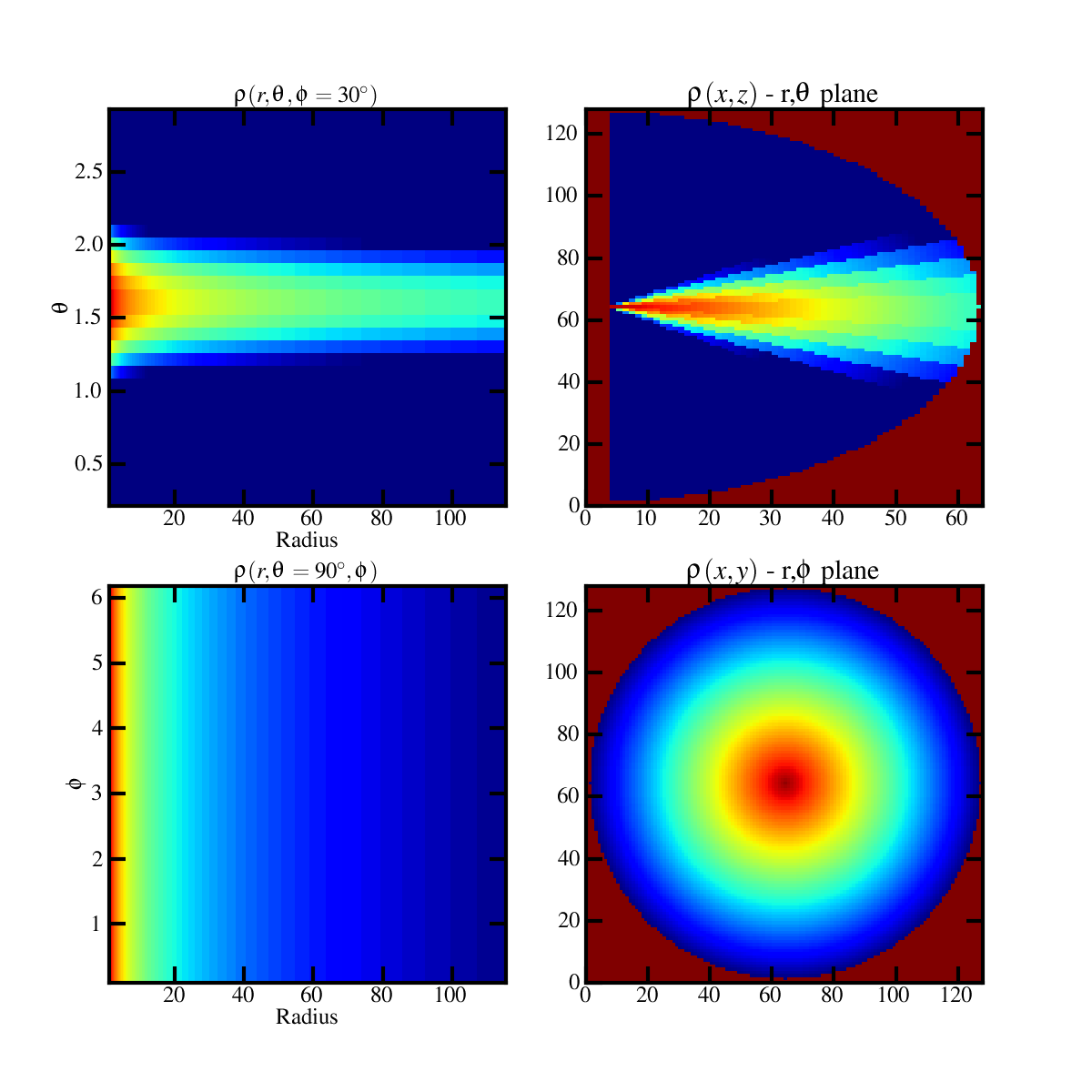# IDL's Polar_surface equivalent in Matplotlib.

Hello Matplotlib Users.

I am trying to work my way out to interpolate a surface from polar coordinates (R,Theta,Z) to rectangular co-ordinates(X,Y,Z)
Basically I am looking for an equivalent for POLAR_SURFACE.pro routine in IDL using matplotlib/Scipy?

http://idlastro.gsfc.nasa.gov/idl_html_help/POLAR_SURFACE.html

Does anyone of you have done that before or could tell me what functions can I use to get that?

Regards
Bhargav Vaidya

(Quick Note: technically, you have cylindrical coordinates because you have Z, not Phi)

While I am sure there are some more optimal methods, the straight-forward way is to do something like the following:

import numpy as np
from scipy.interpolate import griddata

orig_x = Rnp.cos(Theta)
orig_y = R
np.sin(Theta)
orig_z = Z

grid_x, grid_y, grid_z = np.mgrid[orig_x.min():orig_x.max():10j,
orig_y.min():orig_y.max():10j,

``````                                            orig_z.min():orig_z.max():10j]
``````

new_vals = griddata((orig_x, orig_y, orig_z), orig_vals, (grid_x, grid_y, grid_z)

After running this, you should have new_vals which should have the same shape as grid_x. You can also specify the interpolation method used by griddata through the ‘method’ keyword argument. The ‘10j’ in the np.mgrid[] call merely specifies the number of points you want in that axis and can be changed to any other integer you want (just keep the ‘j’ there to make it work the way we want).

I hope that helps!
Ben Root

···

On Wed, Feb 23, 2011 at 2:09 PM, bhargav vaidya <coolastro@…287…> wrote:

Hello Matplotlib Users.

I am trying to work my way out to interpolate a surface from polar coordinates (R,Theta,Z) to rectangular co-ordinates(X,Y,Z)

Basically I am looking for an equivalent for POLAR_SURFACE.pro routine in IDL using matplotlib/Scipy?

http://idlastro.gsfc.nasa.gov/idl_html_help/POLAR_SURFACE.html

Does anyone of you have done that before or could tell me what functions can I use to get that?

Regards

Bhargav Vaidya

Hello Matplotlib Users.

I am trying to work my way out to interpolate a surface from polar coordinates (R,Theta,Z) to rectangular co-ordinates(X,Y,Z)
Basically I am looking for an equivalent for POLAR_SURFACE.pro routine in IDL using matplotlib/Scipy?

http://idlastro.gsfc.nasa.gov/idl_html_help/POLAR_SURFACE.html

Does anyone of you have done that before or could tell me what functions can I use to get that?

Regards
Bhargav Vaidya

(Quick Note: technically, you have cylindrical coordinates because you have Z, not Phi)

While I am sure there are some more optimal methods, the straight-forward way is to do something like the following:

import numpy as np
from scipy.interpolate import griddata

orig_x = Rnp.cos(Theta)
orig_y = R
np.sin(Theta)
orig_z = Z

grid_x, grid_y, grid_z = np.mgrid[orig_x.min():orig_x.max():10j,
orig_y.min():orig_y.max():10j,
orig_z.min():orig_z.max():10j]

new_vals = griddata((orig_x, orig_y, orig_z), orig_vals, (grid_x, grid_y, grid_z)

After running this, you should have new_vals which should have the same shape as grid_x. You can also specify the interpolation method used by griddata through the ‘method’ keyword argument. The ‘10j’ in the np.mgrid[] call merely specifies the number of points you want in that axis and can be changed to any other integer you want (just keep the ‘j’ there to make it work the way we want).

I hope that helps!
Ben Root

Hello Benjamin

Thanks for the reply… I was a little vague in my first question before…

Here is my specific problem

I have a 3D matrix say of density (rho [ r, theta , phi] )

where r, theta ,phi have (64,32,32) elements

theta and phi values are in radians.

I have to interpolate rho(r, theta) at some phi to rho(x,z) and rho(r, phi) at some theta to rho(x,y) …

However I used the a different code then the one you mentioned … I am using the scipy.ndimage.geometric_transform

I am attaching the figure obtained using a code belowTHE PART OF THE CODE TO CONVERT THE POLAR TO CARTESIAN IS GIVEN BELOW

I have the following problems :

1. I am not happy with the interpolation. This is partly because I am using pcolormesh for plotting data as imshow is not working for my irregularly spaced grid. I have also tried higher order for the geometric -transform but the difference is not that appreciable. Can I improve the interpolation is some way?

2. In the right panels of the plot above, I dont get the radial and vertical co-ordinate as the right cartesian values… I think this can be solved if I give the right x y and z values to pcolormesh … but I am not sure how to get the right values for them so that they are consistent with the transform.

import numpy as np

import scipy as S

import scipy.ndimage

def get_polar_plot(self,var,**kwargs):

``````	def r2theta(outcoords, inputshape, origin):

xindex, yindex = outcoords

x0, y0 = origin

x = xindex - x0

y = yindex - y0

r = np.sqrt(x**2 + y**2)

theta = np.arctan2(np.pi-y, x)

theta_index = np.round((theta+ np.pi) * inputshape / (1.015*np.pi))

return (r,theta_index)

def r2phi(outcoords, inputshape, origin):

xindex, yindex = outcoords

x0, y0 = origin

x = xindex - x0

y = yindex - y0

r = np.sqrt(x**2 + y**2)

phi = np.arctan2(y, x)

phi_index = np.round((phi + np.pi) * inputshape / (2.1*np.pi))

return (r,phi_index)

x1 = kwargs.get('x1') # This is Radial co-ordinate

x2 = kwargs.get('x2') # This is Either Theta or Phi

data_arr2d = var

if kwargs.get('rtheta',False) == True:

var_cart = S.ndimage.geometric_transform(data_arr2d, r2theta,order=1,output_shape = (data_arr2d.shape*2, data_arr2d.shape),extra_keywords = {'inputshape':data_arr2d.shape,'origin':(data_arr2d.shape,0)})

if kwargs.get('rphi',False) == True:

var_cart = S.ndimage.geometric_transform(data_arr2d, r2phi, order=1,output_shape = (data_arr2d.shape*2, data_arr2d.shape*2),extra_keywords = {'inputshape':data_arr2d.shape,'origin':(data_arr2d.shape,data_arr2d.shape)})

return var_cart
``````

Hope you will help me in this regard.

Regards

Bhargav

···

On Wed, Feb 23, 2011 at 2:09 PM, bhargav vaidya <coolastro@…287…> wrote: